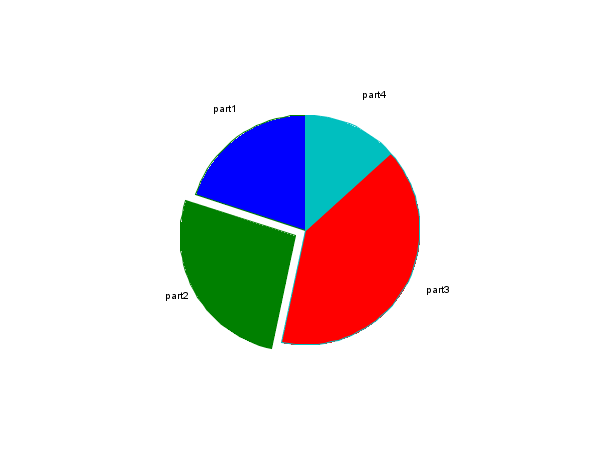Scilab Home page | Wiki | Bug tracker | Forge | Mailing list archives | ATOMS | File exchange
Change language to: Français - Português - 日本語 - Русский

See the recommended documentation of this function

Scilab Help >> Graphics > pie

# pie

draw a pie

### Syntax

```pie(x)
pie(x[,sp[,txt]])```

### Arguments

x

a scalar or a vector of positive reals.

sp

a real scalar or a vector of reals.

txt

a cell or a vector of strings.

### Description

`pie(x):` if size of x is N then pie function draws a pie with N parts, the area of the ith part is equal to (x(i)/sum(x))*( surface of the unit circle).

`pie(x,sp):`the sp vector allows to cut one or several parts of the pie, (the size of sp must be equal to N). if the value of the ith index of sp is different of zero then the ith part is separated from the others by a space, else if it' s equal to zero then it is attached to the others.

`pie(x,txt):` the txt vector allows to write a text for each part of the pie, the ith component of txt corresponds to the ith part (default : it's written the percentages which corresponds to the parts surface). The size of txt must be equal to N.

### Examples

```// First example : one input argument  x=[1 2 5]
scf(0);
pie([1 2 5]);``````// Second example : two input arguments x=[5 9  4 6 3], sp=[0 1 0 1 0], the second and the fourth are separated of the others
scf(1);
pie([5 9 4 6 3],[0 1 0 1 0]);``````// Third example : three input arguments, x=[3 4 6 2], sp=[0 1 0 0], txt=["part1","part2","part3","part4"]
scf(2);
pie([3 4 6 2],[0 1 0 0],["part1","part2","part3","part4"]);```